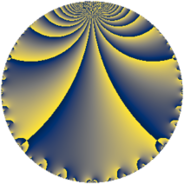# Properties

 Label 28.3.hLevel $28$ Weight $3$ Character orbit 28.h Rep. character $\chi_{28}(5,\cdot)$ Character field $\Q(\zeta_{6})$ Dimension $2$ Newform subspaces $1$ Sturm bound $12$ Trace bound $0$

# Related objects

## Defining parameters

 Level: $$N$$ $$=$$ $$28 = 2^{2} \cdot 7$$ Weight: $$k$$ $$=$$ $$3$$ Character orbit: $$[\chi]$$ $$=$$ 28.h (of order $$6$$ and degree $$2$$) Character conductor: $$\operatorname{cond}(\chi)$$ $$=$$ $$7$$ Character field: $$\Q(\zeta_{6})$$ Newform subspaces: $$1$$ Sturm bound: $$12$$ Trace bound: $$0$$

## Dimensions

The following table gives the dimensions of various subspaces of $$M_{3}(28, [\chi])$$.

Total New Old
Modular forms 22 2 20
Cusp forms 10 2 8
Eisenstein series 12 0 12

## Trace form

 $$2 q + 3 q^{3} + 3 q^{5} - 14 q^{7} - 6 q^{9} + O(q^{10})$$ $$2 q + 3 q^{3} + 3 q^{5} - 14 q^{7} - 6 q^{9} - 15 q^{11} + 6 q^{15} + 51 q^{17} + 27 q^{19} - 21 q^{21} + 9 q^{23} - 22 q^{25} - 12 q^{29} - 21 q^{31} - 45 q^{33} - 21 q^{35} - 31 q^{37} + 24 q^{39} + 20 q^{43} - 18 q^{45} + 75 q^{47} + 98 q^{49} + 51 q^{51} + 57 q^{53} + 54 q^{57} - 141 q^{59} - 141 q^{61} + 42 q^{63} - 24 q^{65} + 49 q^{67} - 252 q^{71} - 45 q^{73} - 66 q^{75} + 105 q^{77} + 73 q^{79} - 9 q^{81} + 102 q^{85} - 18 q^{87} + 99 q^{89} - 21 q^{93} + 27 q^{95} + 180 q^{99} + O(q^{100})$$

## Decomposition of $$S_{3}^{\mathrm{new}}(28, [\chi])$$ into newform subspaces

Label Dim $A$ Field CM Traces $q$-expansion
$a_{2}$ $a_{3}$ $a_{5}$ $a_{7}$
28.3.h.a $2$ $0.763$ $$\Q(\sqrt{-3})$$ None $$0$$ $$3$$ $$3$$ $$-14$$ $$q+(1+\zeta_{6})q^{3}+(2-\zeta_{6})q^{5}-7q^{7}-6\zeta_{6}q^{9}+\cdots$$

## Decomposition of $$S_{3}^{\mathrm{old}}(28, [\chi])$$ into lower level spaces

$$S_{3}^{\mathrm{old}}(28, [\chi]) \cong$$ $$S_{3}^{\mathrm{new}}(14, [\chi])$$$$^{\oplus 2}$$